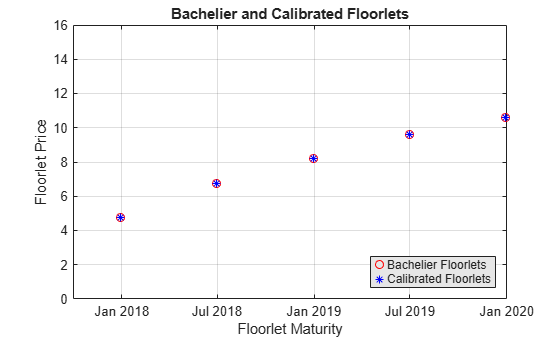# Calibrating Floorlets Using the Normal (Bachelier) Model

This example shows how to use `hwcalbyfloor` to calibrate market data with the Normal (Bachelier) model to price floorlets. Use the Normal (Bachelier) model to perform calibrations when working with negative interest rates, strikes, and normal implied volatilities.

Consider a floor with these parameters:

```Settle = 'Dec-30-2016'; Maturity = 'Dec-30-2019'; Strike = -0.004075; Reset = 2; Principal = 100; Basis = 0;```

The floorlets and market data for this example are defined as:

```floorletDates = cfdates(Settle, Maturity, Reset, Basis); datestr(floorletDates')```
```ans = 6x11 char array '30-Jun-2017' '30-Dec-2017' '30-Jun-2018' '30-Dec-2018' '30-Jun-2019' '30-Dec-2019' ```
```% Market data information MarketStrike = [-0.00595; 0]; MarketMat = {'30-Jun-2017';'30-Dec-2017';'30-Jun-2018'; '30-Dec-2018';'30-Jun-2019'; '30-Dec-2019'}; MarketVol = [0.184 0.2329 0.2398 0.2467 0.2906 0.3348; % First row in table corresponding to Strike 1 0.217 0.2707 0.2760 0.2814 0.3160 0.3508]; % Second row in table corresponding to Strike 2```

Define the `RateSpec` using `intenvset`.

```Rates= [-0.003210;-0.003020;-0.00182;-0.001343;-0.001075]; ValuationDate = 'Dec-30-2016'; EndDates = {'30-Jun-2017';'Dec-30-2017';'30-Jun-2018';'Dec-30-2018';'Dec-30-2019'}; Compounding = 2; Basis = 0; RateSpec = intenvset('ValuationDate', ValuationDate, ... 'StartDates', ValuationDate, 'EndDates', EndDates, ... 'Rates', Rates, 'Compounding', Compounding, 'Basis', Basis);```

Use `hwcalbyfloor` to find values for the volatility parameters `Alpha` and `Sigma` using the Normal (Bachelier) model.

```format short o=optimoptions('lsqnonlin','TolFun',100*eps); warning ('off','fininst:hwcalbycapfloor:NoConverge') [Alpha, Sigma, OptimOut] = hwcalbyfloor(RateSpec, MarketStrike, MarketMat,... MarketVol, Strike, Settle, Maturity, 'Reset', Reset, 'Principal', Principal,... 'Basis', Basis, 'OptimOptions', o, 'model', 'normal')```
```Local minimum possible. lsqnonlin stopped because the size of the current step is less than the value of the step size tolerance. ```
```Alpha = 1.0000e-06 ```
```Sigma = 0.3410 ```
```OptimOut = struct with fields: resnorm: 1.9233e-04 residual: [5x1 double] exitflag: 2 output: [1x1 struct] lambda: [1x1 struct] jacobian: [5x2 double] ```

The `OptimOut.residual` field of the `OptimOut` structure is the optimization residual. This value contains the difference between the Normal (Bachelier) floorlets and those calculated during the optimization. Use the `OptimOut.residual` value to calculate the percentual difference (error) compared to Normal (Bachelier) floorlet prices, and then decide whether the residual is acceptable. There is almost always some residual, so decide if it is acceptable to parameterize the market with a single value of `Alpha` and `Sigma`.

Price the floorlets using the market data and Normal (Bachelier) model to obtain the reference floorlet values. To determine the effectiveness of the optimization, calculate reference floorlet values using the Normal (Bachelier) formula and the market data. Note, you must first interpolate the market data to obtain the floorlets for calculation.

```MarketMatNum = datenum(MarketMat); [Mats, Strikes] = meshgrid(MarketMatNum, MarketStrike); FlatVol = interp2(Mats, Strikes, MarketVol, datenum(Maturity), Strike, 'spline'); [FloorPrice, Floorlets] = floorbynormal(RateSpec, Strike, Settle, Maturity, FlatVol,... 'Reset', Reset, 'Basis', Basis, 'Principal', Principal); Floorlets = Floorlets(2:end)'```
```Floorlets = 5×1 4.7637 6.7180 8.1833 9.5825 10.6090 ```

Compare the optimized values and Normal (Bachelier) values, and display the results graphically. After calculating the reference values for the floorlets, compare the values analytically and graphically to determine whether the calculated single values of `Alpha` and `Sigma` provide an adequate approximation.

```OptimFloorlets = Floorlets+OptimOut.residual; disp(' ');```
``` ```
`disp(' Bachelier Calibrated Floorlets');`
``` Bachelier Calibrated Floorlets ```
`disp([Floorlets OptimFloorlets])`
``` 4.7637 4.7685 6.7180 6.7263 8.1833 8.1878 9.5825 9.5795 10.6090 10.6007 ```
```plot(MarketMatNum(2:end), Floorlets, 'or', MarketMatNum(2:end), OptimFloorlets, '*b'); datetick('x', 2) xlabel('Floorlet Maturity'); ylabel('Floorlet Price'); ylim ([0 16]); title('Bachelier and Calibrated Floorlets'); h = legend('Bachelier Floorlets', 'Calibrated Floorlets'); set(h, 'color', [0.9 0.9 0.9]); set(h, 'Location', 'SouthEast'); set(gcf, 'NumberTitle', 'off') grid on```